Exit Ticket for Day 19 of Calculus
Please select your name from this list: *
1. What is the derivative of the following function?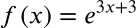2. What is the derivative of the following function?3. What is the derivative of the following function?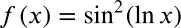4. What is the derivative of the following function?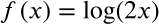5. What is the derivative of this function?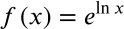6. What is the derivative of this function?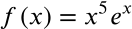This form was created inside of Evansville Vanderburgh School Corporation. Report Abuse - Terms of Service